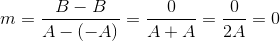# ISEE Middle Level Quantitative : How to find a line on a coordinate plane

## Example Questions

### Example Question #1 : Geometry

Give the equation of the line through point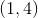that has slope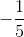.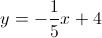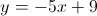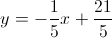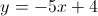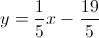Explanation:

Use the point-slope formula with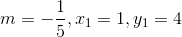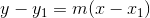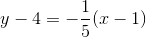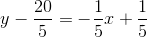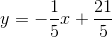### Example Question #2 : Coordinate Geometry

Which is the greater quantity?

(A) The slope of the line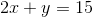(B) The slope of the line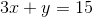(A) is greater

(A) and (B) are equal

(B) is greater

It is impossible to determine which is greater from the information given

(A) is greater

Explanation:

Rewrite each in the slope-intercept form,will be the slope.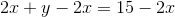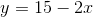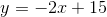The slope of this line is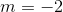.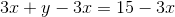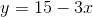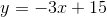The slope of this line is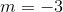.

Since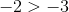, (A) is greater.

### Example Question #3 : Coordinate Geometry

Which is the greater quantity?

(A) The slope of the line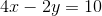(B) The slope of the line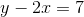(A) and (B) are equal

It is impossible to determine which is greater from the information given

(B) is greater

(A) is greater

(A) and (B) are equal

Explanation:

Rewrite each in the slope-intercept form,will be the slope.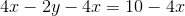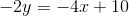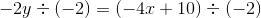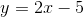The slope of the line ofis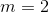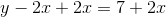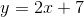The slope of the line ofis alsoThe slopes are equal.

### Example Question #4 : Coordinate Geometryandare positive integers, and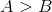. Which is the greater quantity?

(a) The slope of the line on the coordinate plane through the points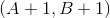and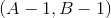.

(b) The slope of the line on the coordinate plane through the points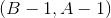and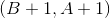.

(a) and (b) are equal

(a) is the greater quantity

It is impossible to determine which is greater from the information given

(b) is the greater quantity

(a) and (b) are equal

Explanation:

The slope of a line through the pointsandcan be found by setting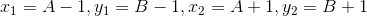in the slope formula: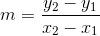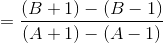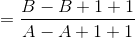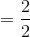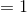The slope of a line through the pointsandcan be found similarly: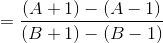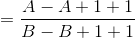The lines have the same slope.

### Example Question #5 : Coordinate Geometry

A line passes through the points with coordinates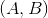and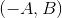, where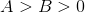. Which expression is equal to the slope of the line?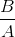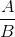UndefinedExplanation:

The slope of a line through the pointsand, can be found by setting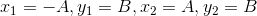:

in the slope formula: